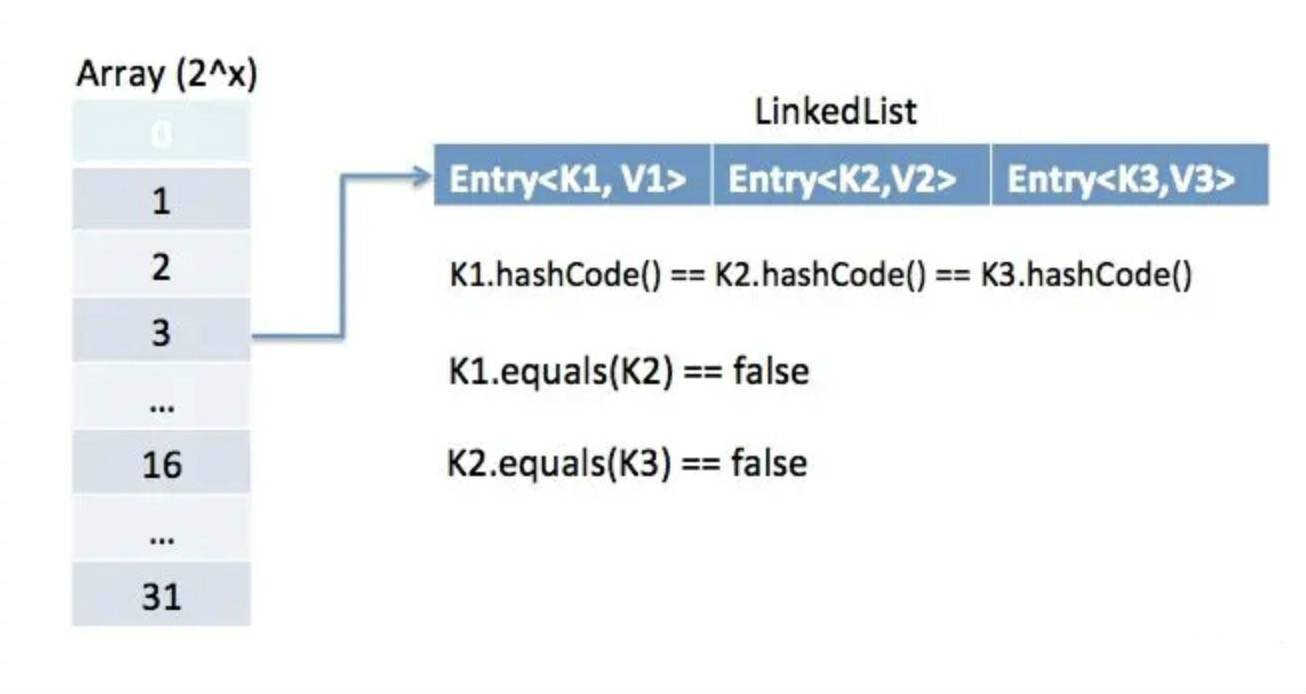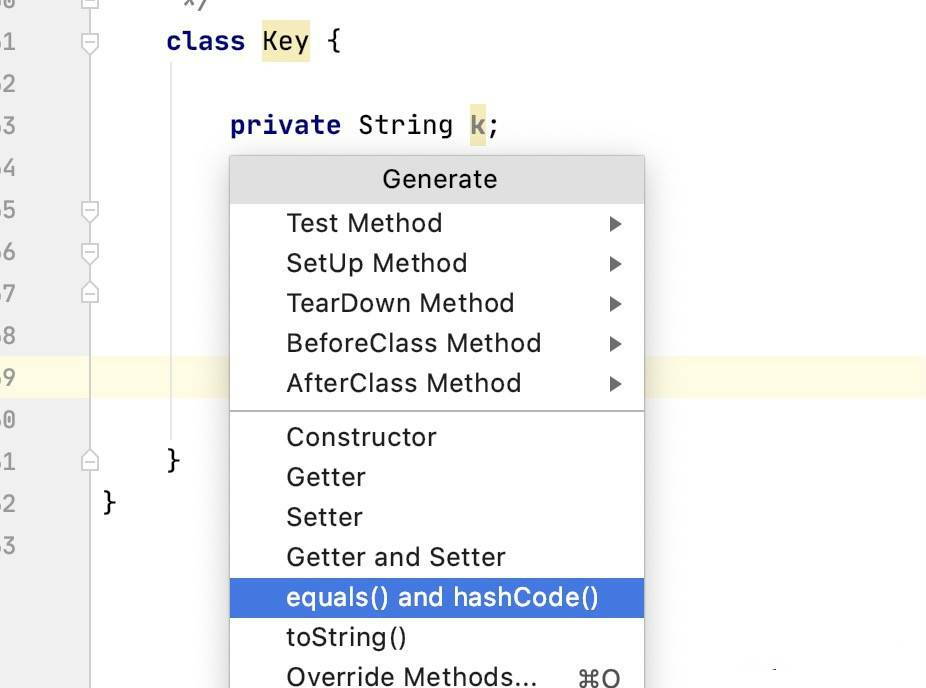Java面试题：重写了equals，还要重写hashCode？

回顾equals方法

public boolean equals(Object obj) {
return (this == obj);
}

与hashCode方法的关系

Note that it is generally necessary to override the hashCode method whenever this method is overridden, so as to maintain the general contract for the hashCode method, which states that equal objects must have equal hash codes.

hashCode方法约定

（1）如果对象在使用equals方法中进行比较的参数没有修改，那么多次调用一个对象的hashCode()方法返回的哈希值应该是相同的。

（2）如果两个对象通过equals方法比较是相等的，那么要求这两个对象的hashCode方法返回的值也应该是相等的。

（3）如果两个对象通过equals方法比较是不同的，那么也不要求这两个对象的hashCode方法返回的值是不相同的。但是我们应该知道对于不同对象产生不同的哈希值对于哈希表(HashMap等)能够提高性能。

hashCode应用场景Object中该方法是一个本地方法，会返回一个int类型的哈希值。可以通过将对象的内部地址转换为整数来实现的，但是Java中没有强制要求通过该方式实现。

public void test1() {
String s = "ok";
StringBuilder sb = new StringBuilder(s);
System.out.println(s.hashCode() + "  " + sb.hashCode());

String t = new String("ok");
StringBuilder tb = new StringBuilder(s);
System.out.println(t.hashCode() + "  " + tb.hashCode());
}

3548  1833638914
3548  1620303253

String实现了hashCode方法，而StringBuilder并没有实现，这就导致即使值是一样的，hashCode也不同。

@Test
public void test2() {
String hello = "hello";

Map<String, String> map1 = new HashMap<>();
String s1 = new String("key");
String s2 = new String("key");
map1.put(s1, hello);
System.out.println("s1.equals(s2):" + s1.equals(s2));
System.out.println("map1.get(s1):" + map1.get(s1));
System.out.println("map1.get(s2):" + map1.get(s2));

Map<Key, String> map2 = new HashMap<>();
Key k1 = new Key("A");
Key k2 = new Key("A");
map2.put(k1, hello);
System.out.println("k1.equals(k2):" + s1.equals(s2));
System.out.println("map2.get(k1):" + map2.get(k1));
System.out.println("map2.get(k2):" + map2.get(k2));
}

class Key {

private String k;

public Key(String key) {
this.k = key;
}

@Override
public boolean equals(Object obj) {
if (obj instanceof Key) {
Key key = (Key) obj;
return k.equals(key.k);
}
return false;
}
}

s1.equals(s2):true
map1.get(s1):hello
map1.get(s2):hello
k1.equals(k2):true
map2.get(k1):hello
map2.get(k2):null

@Override
public int hashCode(){
return k.hashCode();
}

s1.equals(s2):true
map1.get(s1):hello
map1.get(s2):hello
k1.equals(k2):true
map2.get(k1):hello
map2.get(k2):hello

public V put(K key, V value) {
return putVal(hash(key), key, value, false, true);
}

static final int hash(Object key) {
int h;
return (key == null) ? 0 : (h = key.hashCode()) ^ (h >>> 16);
}

final V putVal(int hash, K key, V value, boolean onlyIfAbsent,
boolean evict) {
Node<K,V>[] tab; Node<K,V> p; int n, i;
if ((tab = table) == null || (n = tab.length) == 0)
n = (tab = resize()).length;
// 通过哈希值来查找底层数组位于该位置的元素p，如果p不为null，则使用新的键值对来覆盖旧的键值对
if ((p = tab[i = (n - 1) & hash]) == null)
tab[i] = newNode(hash, key, value, null);
else {
Node<K,V> e; K k;
// (二者哈希值相等)且(二者地址值相等或调用equals认定相等)。
if (p.hash == hash &&
((k = p.key) == key || (key != null && key.equals(k))))
e = p;
else if (p instanceof TreeNode)
e = ((TreeNode<K,V>)p).putTreeVal(this, tab, hash, key, value);
else {
for (int binCount = 0; ; ++binCount) {
if ((e = p.next) == null) {
p.next = newNode(hash, key, value, null);
if (binCount >= TREEIFY_THRESHOLD - 1) // -1 for 1st
treeifyBin(tab, hash);
break;
}
if (e.hash == hash &&
((k = e.key) == key || (key != null && key.equals(k))))
break;
p = e;
}
}
// 如果底层数组中存在传入的Key，那么使用新传入的覆盖掉查到的
if (e != null) { // existing mapping for key
V oldValue = e.value;
if (!onlyIfAbsent || oldValue == null)
e.value = value;
afterNodeAccess(e);
return oldValue;
}
}
++modCount;
if (++size > threshold)
resize();
afterNodeInsertion(evict);
return null;
}

重写hashCode方法@Override
public boolean equals(Object o) {
if (this == o) {
return true;
}
if (o == null || getClass() != o.getClass()) {
return false;
}
Key key = (Key) o;
return Objects.equals(k, key.k);
}

@Override
public int hashCode() {
return Objects.hash(k);
}

public static int hash(Object... values) {
return Arrays.hashCode(values);
}

public static int hashCode(Object a[]) {
if (a == null)
return 0;

int result = 1;

for (Object element : a)
result = 31 * result + (element == null ? 0 : element.hashCode());

return result;
}

public static int hashCode(Object o) {
return o != null ? o.hashCode() : 0;
}

0 人点赞